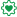# New to Qlik Sense

Discussion board where members can get started with Qlik Sense.

Announcements
Coming your way, the Qlik Data Revolution Virtual Summit. October 27-29. REGISTER
cancel
Showing results for
Did you mean:
HighlightedEmployee

## Need to ignore one filter

I can not figure out where to place the set analysis in this expression to ignore a single filter (FISCAL_YEAR).  It currently has no Set Analysis and every place I have tried to put it throws and error.

{<FISCAL_YEAR=>}

I'm not sure I can even do it since the expression is actually using this field.

='Total Realized Impact: ' & NUM(Sum (if(FISCAL_YEAR =If(num(Month(Now())) <= 6, Year(Now()) - 1, Year(Now())), MONTHLY_QUANTIFIED)), '\$ #,##0','.',',') &'
Total Target Impact: ' & NUM(Sum (if(FISCAL_YEAR = If(num(Month(Now())) <= 6, Year(Now()) - 1, Year(Now())), MONTHLY_TARGET)), '\$ #,##0','.',',')

Any guidance is greatly appreciated.

1 Solution

Accepted Solutions
HighlightedPartner

declare variable like so vYear = If(num(Month(Now())) <= 6, Year(Now()) - 1, Year(Now()) )

Sum ( {<FISCAL_YEAR ={"\$(=vYear)"}>} MONTHLY_QUANTIFIED)

2 Replies
HighlightedPartner

declare variable like so vYear = If(num(Month(Now())) <= 6, Year(Now()) - 1, Year(Now()) )

Sum ( {<FISCAL_YEAR ={"\$(=vYear)"}>} MONTHLY_QUANTIFIED)

HighlightedContributor III

I am not sure about the logic of the calculation but that's how you ignore filters - if you had a column, called 'FromDate' and you want to count IDs, ignoring any filters applied to it, then this will be the expression:

Count({<FromDate=>}ID)

My advice - build up the formula step by step so you can figure out where the error is coming from, it could be an extra bracket or the right number of brackets but in the wrong places.